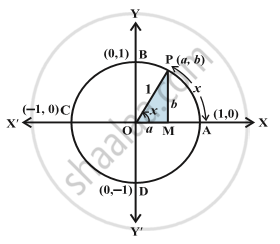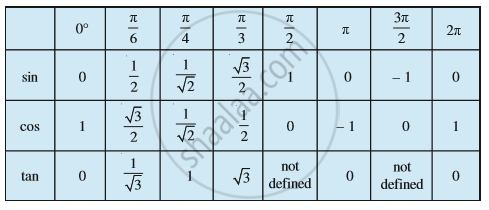# Introduction of Trigonometric Functions

#### description

• Trigonometric Functions with the Help of Unit Circle

#### notes

In earlier classes, we have studied trigonometric ratios for acute angles as the ratio of sides of a right angled triangle. We will now extend the definition of trigonometric ratios to any angle in terms of radian measure and study them as trigonometric functions. Consider a unit circle with centre at origin of the coordinate axes. Let P (a, b) be any point on the circle with angle AOP = x radian, i.e., length of arc AP = x.The radius OA keeps on moving, at poin A the angle is 0. At point B the will be π/2, at point C it will be π, at point D it will be (3π)/2 and after completing one rotation coming back to point A the angle will be 2π.
If a radius r forms a angle θ then the coordinates at that particular points will be (r cosθ, r sinθ)
Let x= θ
Thus, at P a= cos x and b= sin x
Similarly, at A the corordinates are (cos 0, sin 0)
at B the corordinates are (cos 90, sin 90)
at C the corordinates are (cos π, sin π)
at D the corordinates are (cos (3π)/2, sin (3π)/2)
And after completing one rotation coming back to point A, the corordinates are (cos 2π, sin 2π)
On x axis, sinθ= 0 implies θ= nπ, where n is any integer
On y  "axis", cosθ= 0 implies θ= (2n+1)  π/2, where n is any integer.
In earlier classes, we have discussed the values of trigonometric ratios for 0°, 30°, 45°, 60° and 90°. The values of trigonometric functions for these angles are same as that of trigonometric ratios studied in earlier classes. Thus, we have the following table:If you would like to contribute notes or other learning material, please submit them using the button below.